# NCERT Solutions for Class 8 Maths Chapter 5 Data Handling Ex 5.2

NCERT Solutions for Class 8 Maths Chapter 5 Data Handling Ex 5.2

### NCERT Solutions for Class 8 Maths Chapter 5 Data Handling Exercise 5.2

Ex 5.2 Class 8 Maths Question 1.
A survey was made to find the type of music that a certain group of young people liked in a city. The adjoining pie chart shows the findings of this survey.From this pie chart answer the following:Ex 5.2 Class 8 Maths Question 2.
A group of 360 people were asked to vote for their favourite season from the three seasons rainy, winter and summer.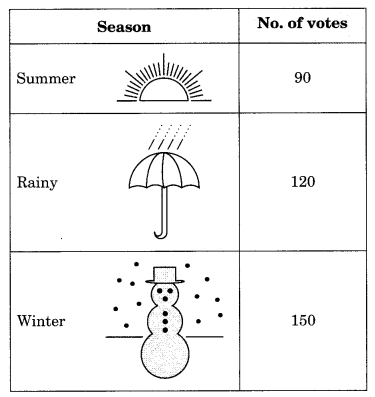(i) Which season got the most votes?
(ii) Find the central angle of each sector.
(iii) Draw a pie chart to show this information.
Solution:
(i) Winter season got the most votes, i.e. 150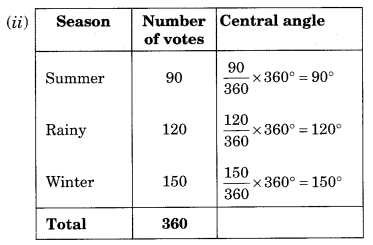(iii) Pie chart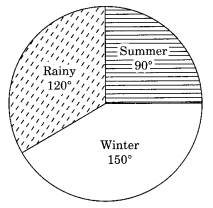Ex 5.2 Class 8 Maths Question 3.
Draw a pie chart showing the following information. The table shows the colours preferred by a group of people.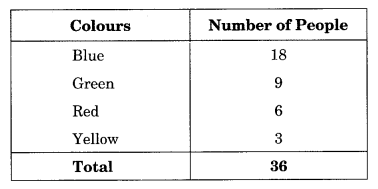Solution:
Table to find the central angle of each sector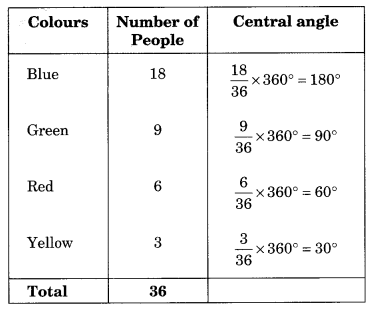Ex 5.2 Class 8 Maths Question 4.
The following pie chart gives the marks scored in an examination by a student in Hindi, English, Mathematics, Social Science and Science. If the total marks obtained by the students were 540, answer the following questions.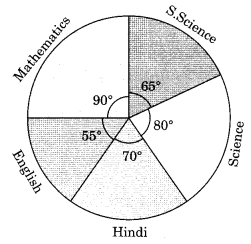(i) In which subject did the student score 105 marks?
(Hint: for 540 marks, the central angle = 360°. So, for 105 marks, what is the central angle?)
(ii) How many more marks were obtained by the student in Mathematics than in Hindi?
(iii) Examine whether the sum of the marks obtained in Social Science and Mathematics is more than that in Science and Hindi.
(Hint: Just study the central angles).
Solution: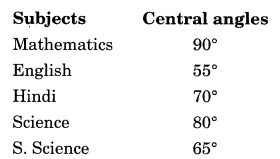Ex 5.2 Class 8 Maths Question 5.
Marks obtained in Social Science and Mathematics are more than that of the marks obtained in Science and Hindi.
The number of students in a hostel, speaking different languages is given below. Display the data in a pie chart.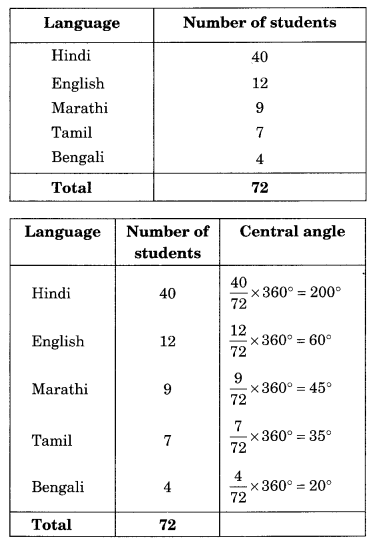Solution: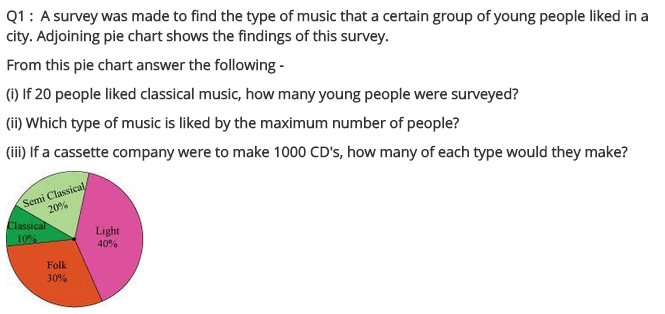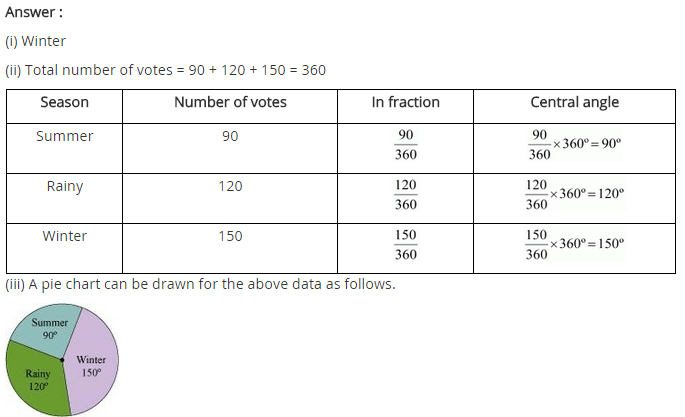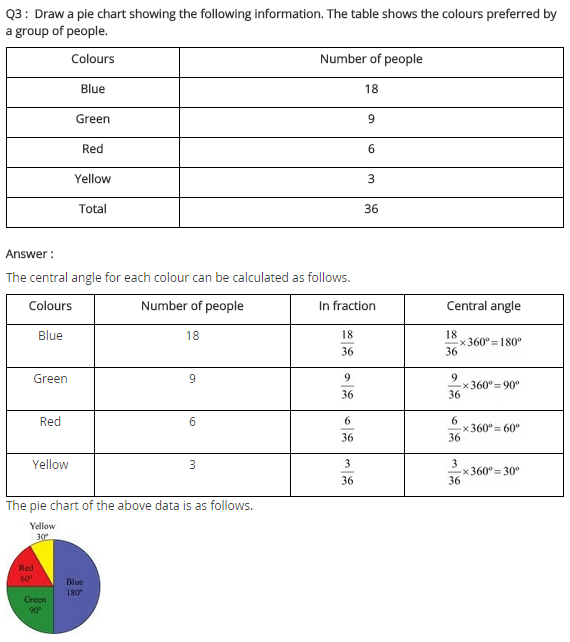## SabDekho

The Complete Educational Website